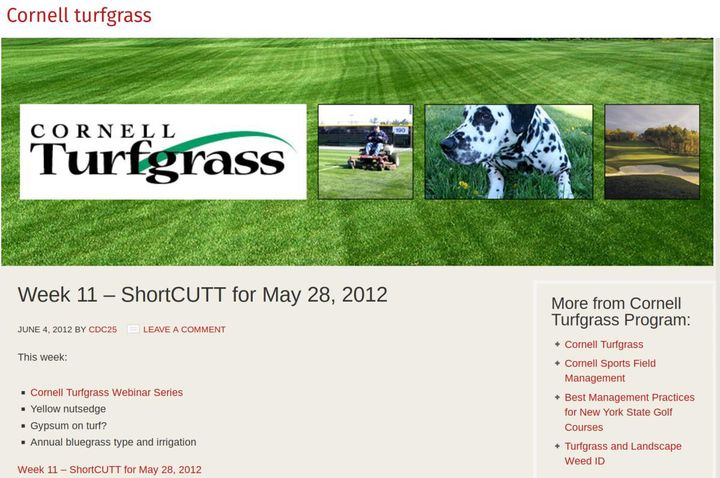# Calcium deficiency in turfgrass, an imaginary problem?The inimitable Dr. Frank Rossi from Cornell University spoke about supplemental calcium for turfgrass in the 28 May 2012 ShortCUTT podcast, saying it is time to stop worrying about “imaginary problems.”

So is a shortage of calcium really an imaginary problem? Yes.

Let’s make a few calculations to show why.

Actively growing putting green turf may produce about 100 g of dry matter per square meter harvested as clippings each month.

If the dried clippings contain 0.5% calcium, a more than sufficient amount, that is a monthly harvest of 0.5 g calcium per square meter per month. That is what the grass is using. And that is how much calcium the soil must supply.

Calcium, of course, is primarily supplied to the roots by mass flow, moving to the roots with water, dissolved in the soil solution. The water use of the grass can be estimated by evapotranspiration (ET). The ET varies with grass type and weather conditions, but let’s assume the ET averages 5 mm per day. That is equivalent to 5 L of water used per day per square meter of turf.

With 5 L of water per day, and 30 days in a month, we have 150 L of water used per month, and that water needs to contain at least 0.5 g of calcium to supply what is harvested through clipping removal. What is that concentration then, in soil solution? It is 0.5 g / 150 L = 500 mg / 150 L = 3.3 ppm. What is the typical range of calcium concentration in soil solutions? About 40 to 400 ppm. So there is typically a much higher (by a factor of about 10) level of calcium in soil solution than the grass requires.

That is just one of many reasons why Dr. Rossi can call calcium deficiencies or perceived shortages of calcium an imaginary problem.